Latex Support

LaTeX has been integrated into this site, making it easy to include mathematics in the pages you edit. This is not a standard feature of PmWiki, so it is not covered in the standard documentation on text formatting rules. This page explains how to include LaTeX code in the pages you edit and addresses some complications that arise.

You can find more explanations in Original Latex Support (thanks for the LaTeX engine to Joe Miller, and his now defunct Effective Randomness wiki). You can find additional features not necessary about LaTeX in Advanced Formatting.

The syntax used to produce LaTeX input is the same as what you would expect. For example, the formulais produced by the code $A\in 2^\omega$. The usual markup is also provided for display mathematics.

Here some examples of formulas are presented. Press Edit button to see the code.

1. $\sqrt{2^\frac{1}{x^2+1}+1}$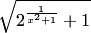2. $A=\left(\begin{array}{cc} 1 & 2 \\ 3 & 4 \end{array}\right)$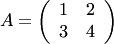3. For all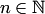: $$\sum_{i=0}^n i=\frac{n(n+1)}{2}$$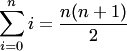4. Integral calculus: $$\int_0^{+\infty} \frac{\sin t}{t}\, dt=\frac{\pi}{2}$$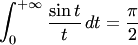5. Inline syntax: $$a^2=b$$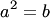$c=d+d$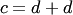6. Begin/end syntax: \begin{align*} x_k&=Ax_{k-1}+Bu_k+w_k,\\ z_k&=Hx_k+v_k, \end{align*}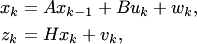Important: The begin/end syntax can only be used for formulas that fit one line in the source file (although they may occupy more than one line after compilation).

Some formulas do not work:

1. $a^{(N)}$ provides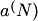. But you can always use $a^{\left(N\right)}$, which provides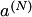, or $a^{\:(N)\:}$ provides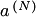.
2. $[-Y,Y]$ is not handled well. But you can always use $[-Y,Y]$, which provides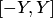.